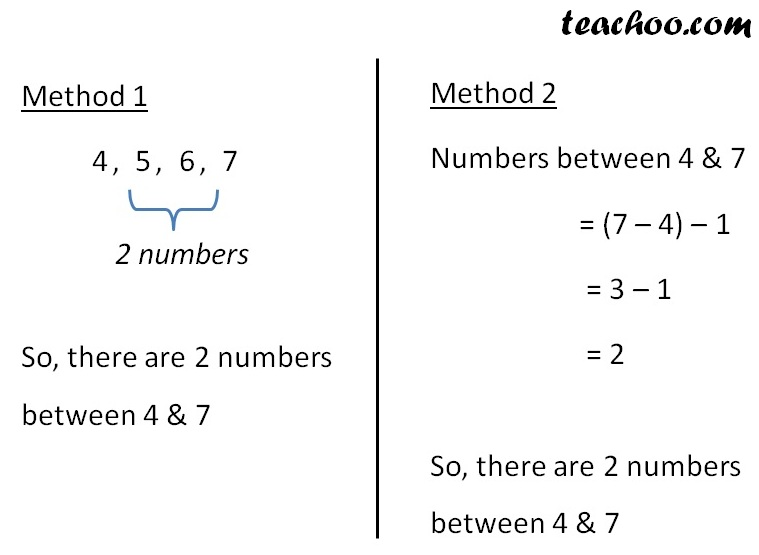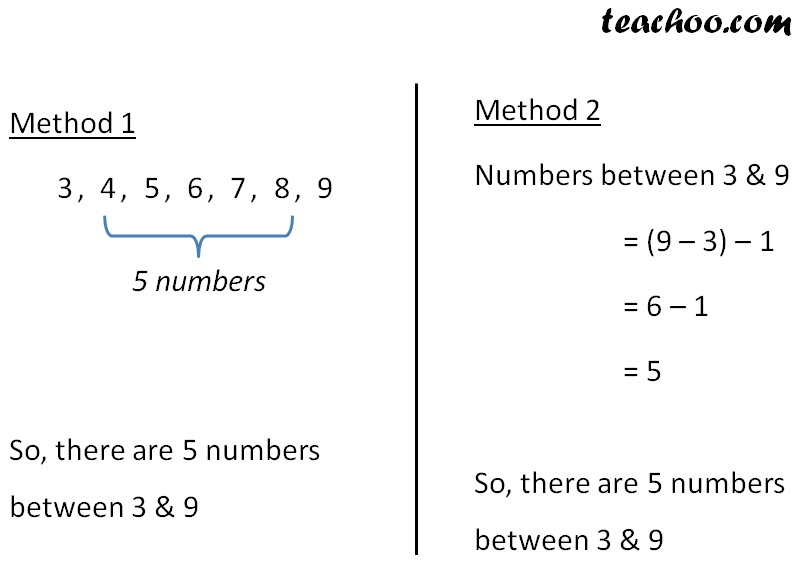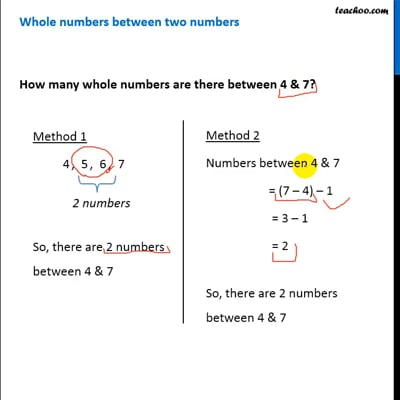Whole number between two numbers

Chapter 2 Class 6 Whole Numbers
Concept wise

## How many whole numbers are there between 4 & 7?## How many whole numbers are there between 3 & 9?This video is only available for Teachoo black users

### Transcript

Whole numbers between two numbers Method 1 4, 5, 6, 7 So, there are 2 numbers between 4 & 7 Method 2 Numbers between 4 & 7 = (7 – 4) – 1 = 3 – 1 = 2 So, there are 2 numbers between 4 & 7 Method 2 Numbers between 4 & 7 = (7 – 4) – 1 = 3 – 1 = 2 So, there are 2 numbers between 4 & 7 How many whole numbers are there between 3 & 9? Method 1 3, 4, 5, 6, 7, 8, 9 So, there are 5 numbers between 3 & 9 Method 2 Numbers between 3 & 9 = (9 – 3) – 1 = 6 – 1 = 5 So, there are 5 numbers between 3 & 9